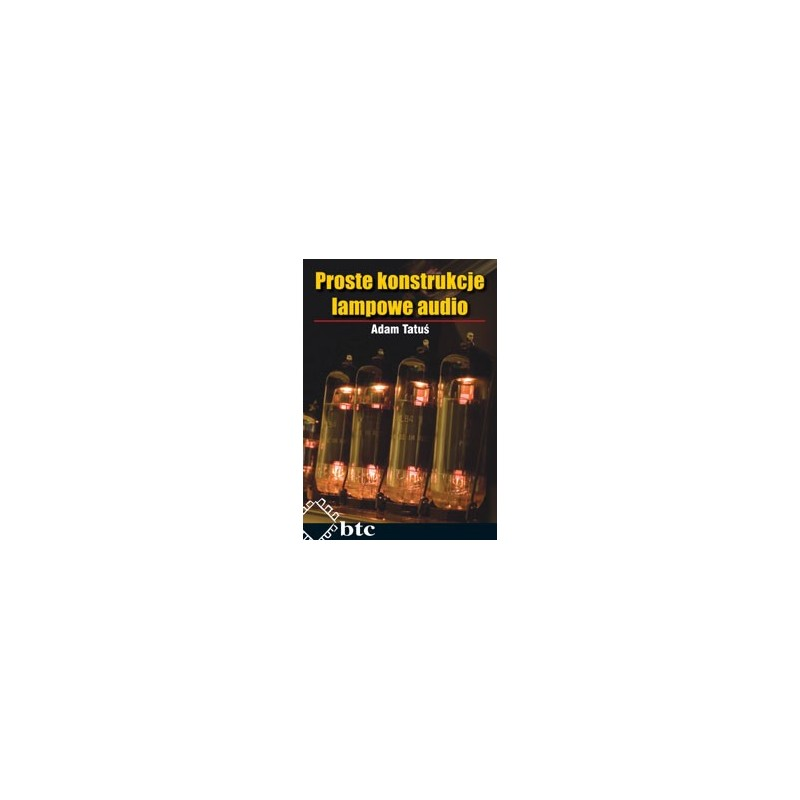﻿
Obniżka!Zobacz większe

# Handbook of Differential Equations: Stationary Partial Differential Equations

Michel Chipot

ID: 172664

### Więcej informacji

This handbook is the sixth and last volume in the series devoted to stationary partial differential equations. The topics covered by this volume include in particular domain perturbations for boundary value problems, singular solutions of semi-linear elliptic problems, positive solutions to elliptic equations on unbounded domains, symmetry of solutions, stationary compressible Navier-Stokes equation, Lotka-Volterra systems with cross-diffusion, fixed point theory for elliptic boundary value problems.

* Collection of self contained, state-of-the-art surveys
* Written by well-known experts in the field
* Informs and updates on all the latest developments

1. Domain Perturbation for Linear and Semi-Linear Boundary Value Problems, D. Daners.
2. Singular solutions of semi-linear elliptic problems J. Dávila.
3. Positive solutions to semi- linear and quasi-linear elliptic equations on unbounded domains, V. Kondratiev, V. Liskevich and Z. Sobol.
4. Symmetry of solutions of elliptic equations via maximum principles, F. Pacella and M. Ramaswamy.
5. Stationary Boundary Value Problems for Compressible Navier-Stokes equations, P.I. Plotnikov and J. Sokolowski.
6. Positive Solutions for Lotka-Volterra Systems with Cross- Diffusion, Y. Yamada.
7. Fixed Point Theory and Elliptic Boundary Value Problems, H. Zou.